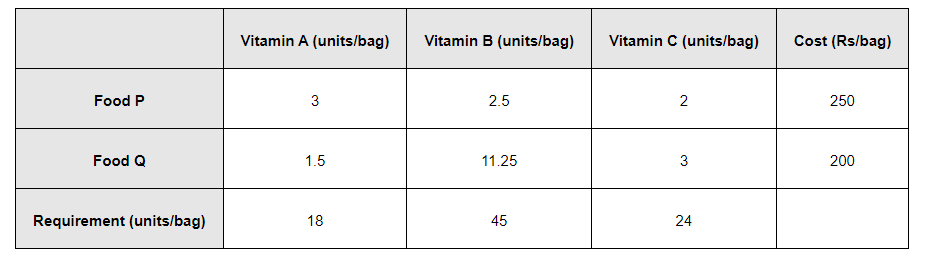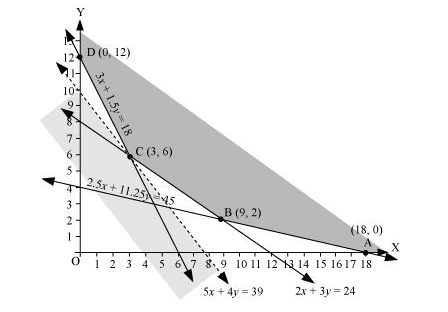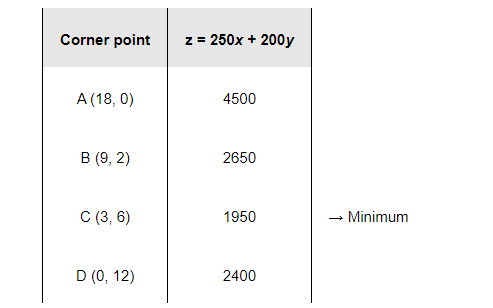# A farmer mixes two brands P and Q of cattle feed. Brand P,

Question:

A farmer mixes two brands P and Q of cattle feed. Brand P, costing Rs 250 per bag contains 3 units of nutritional element A, 2.5 units of element B and 2 units of element C. Brand Q costing Rs 200 per bag contains 1.5 units of nutritional elements A, 11.25 units of element B, and 3 units of element C. The minimum requirements of nutrients A, B and C are 18 units, 45 units and 24 units respectively. Determine the number of bags of each brand which should be mixed in order to produce a mixture having a minimum cost per bag? What is the minimum cost of the mixture per bag?

Solution:

Let the farmer mix x bags of brand P and y bags of brand Q.

The given information can be compiled in a table as follows.The given problem can be formulated as follows.

Minimize $z=250 x+200 y$.           (1)

subject to the constraints,

$3 x+1.5 y \geq 18$                       (2)

$2.5 x+11.25 y \geq 45$                (3)

$2 x+3 y \geq 24$                         (4)

$x, y \geq 0$                                 (5)

The feasible region determined by the system of constraints is as follows.The corner points of the feasible region are A (18, 0), B (9, 2), C (3, 6), and D (0, 12).

The values of z at these corner points are as follows.As the feasible region is unbounded, therefore, 1950 may or may not be the minimum value of z.

For this, we draw a graph of the inequality, $250 x+200 y<1950$ or $5 x+4 y<39$, and check whether the resulting half plane has points in common with the feasible region or not.

It can be seen that the feasible region has no common point with $5 x+4 y<39$

Therefore, the minimum value of z is 1950 at (3, 6).

Thus, 3 bags of brand P and 6 bags of brand Q should be used in the mixture to minimize the cost to Rs 1950.